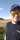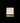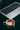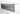Navigate back to the homepage

# Computing relative dates in pandasJuho Salli
May 1st, 2020 · 1 min readHero image credits to Charles Deluvio on Unsplash.

## Computing relative dates in pandas

I had to compute the relative differences of dates based by some other column value.

My first thought was that of course there’s a built in functionality for this in pandas, but to my surprise I couldn’t find any.

I came up with this solution.

## Solution

The full notebook can be found here.

First, let’s create a DataFrame with same dates and IDs. (I’ll use the ID-values to group the dates.)

`.css-1chxjt6{position:absolute;right:22px;top:24px;padding:8px 12px 7px;border-radius:5px;color:#6f7177;-webkit-transition:background 0.3s ease;transition:background 0.3s ease;}.css-1chxjt6:hover{background:rgba(255,255,255,0.07);}.css-1chxjt6[data-a11y="true"]:focus::after{content:"";position:absolute;left:-2%;top:-2%;width:104%;height:104%;border:2px solid var(--theme-ui-colors-accent,#6166DC);border-radius:5px;background:rgba(255,255,255,0.01);}@media (max-width:45.9375em){.css-1chxjt6{display:none;}}1import pandas as pd2import numpy as np3from pandas.util.testing import makeTimeDataFrame45# Dummy dataframe with date as index6df = makeTimeDataFrame()78# Add a random ID-column9df['ID'] = np.random.randint(1, 4, df.shape)10# Drop unnecessary columns11df = df.drop(['A', 'B', 'C', 'D'], axis = 1)1213# put the date in it's own column14df['date'] = df.index1516# reset the index17df = df.reset_index(drop = True)`

Then the data needs to be sorted for the solution to work correctly.

`1# sort the dataframe by the date-column2df = df.sort_values(by = ['date'])`

The dataframe now looks like this:The function `do_date_differences` is the one that does the computing.

`1# row is the target row2# col is the name of the column in the row that I want to "group by"3# date_col is the name of the column that has the date-formatted data4def do_date_differences(row, col, date_col = 'date'):5    first = df.loc[df[col].isin([row[col]])].iloc[date_col]6    second = row[date_col]7    diff = second - first8    return diff`

The function works by finding out the first date occurence of the value in the given (grouper) column for the target row. Then it calculates the difference of the found (first) date and the date that’s in the date_col of the target row. The difference is returned so it can be added as a new column value to the row.

And then by using the dataframe’s apply-function, call the do_date_differences.

`1# I want to find relative dates for all the rows in the dataframe, grouped by the ID-column2df['difference'] = df.apply(lambda row: do_date_differences(row, 'ID', 'date'), axis=1)`

The dataframe now looks like this:-Juho

### More articles from Juho Salli### From bug to fix to production

Sometimes finding the fix for a bug is fast.

August 30th, 2019 · 1 min read### Getting CSS styles in a web page

Getting CSS styles in a web page

June 27th, 2019 · 1 min read